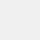# Cymath - Math Problem Solver

Version 2.45
 Install +5 K From 30 Rates 4.1 Category Education Size 6 MB Last Update 2023 November 4# Cymath - Math Problem Solver

Cymath LLC
Version 2.45
 Install +5 K From 30 Rates 4.1 Category Education Size 6 MB Last Update 2023 November 4
View the antivirus scan results

## Introduction

With millions of Cymath.com users worldwide, the Cymath math problem solver app uses the same math engine while letting you solve problems on the go! Just enter a problem from your math homework, and let Cymath solve it for you step-by-step! We provide algebra as well as calculus help.

Topics in algebra include: equation solving, factoring, logarithms, exponents, complex numbers, quadratic equations, trigonometry, partial fraction, polynomial division, etc. Topics in calculus include: product rule, quotient rule, chain rule, u-substitution, integration by parts, integration by partial fraction, trigonometric substitution, rationalizing substitution, and much more. We provide math answers and steps for all of the above.

In short, whether you are simply stuck on your math homework, want to check your homework answers, or need specific calculus help, let our math problem solver help now!

User Reviews - 30 Rates
4.1 from 5
5
4
3
2
1good boy
2022/03/29
برای امتحانای آنلاین کارامد بود😂AMIR
2022/02/15
عالیArmita
2021/05/21
میشه بگید چطوری کار میکنه اگه سریع جوابمو بدین ممنون میشم برای امتحانم میخوام